﻿ Introduction to Exponential Functions

#Introduction to Exponential Functions

You may also want to review basic properties of logarithmic functions:  Introduction to Logarithmic Functions

Most of your experience thus far has likely been with functions where the base is a variable, and the exponent is a constant, like these:

• $\,y=x=x^1\,$  (the identity function)
• $\,y=x^2\,$  (the squaring function)
• $\,y=x^3\,$  (the cubing function)
• $\,y=\sqrt{x}=x^{1/2}\,$  (the square root function)
• $\,y=\frac{1}{x}=x^{-1}\,$  (the reciprocal function)

Functions with a variable base and a constant exponent are called power functions.

Switch the role of variable and constant and you get an entirely new family of functions, called exponential functions, which are the subject of this section. For example, these are all exponential functions:

• $y=2^x$
• $y={(\frac{1}{2})}^x$
• $\,y={\text{e}}^x$

The precise definition follows:

DEFINITION exponential function

An exponential function is a function of the form $\,y=b^x\,,$ where $\,b\,$ is a positive number not equal to $\,1\,,$ and $\,x\,$ is any real number.

Thus, exponential functions have a constant base the variable is in the exponent.

The number $\,b\,$ is called the base of the exponential function.

The most important exponential function is when the base is the irrational number $\,\text{e}\,.$ (Note: $\text{e}\approx 2.71828\,$) In this case, the function is also written as $\ \exp(x)\,,$ and is called the natural exponential function.

If you hear the phrase ‘the exponential function’ (meaning only one) then the function being referred to is $\,y={\text{e}}^x=\exp(x)\,.$

Some mathematicians (this author included) tend to call more general functions with a constant base and variable in the exponent exponential functions; say, $\ y={\text{e}}^{2x-3}\$ or $\ y=-7\cdot 3^{5-x}\,.$ However, for the purposes of this section, the phrase exponential function refers only to functions of the form $\,y=b^x\,.$

Why must the base of an exponential function be positive? For negative numbers, there are problems for many values of $\,x\,$:  for example, $\,(-4)^{1/2} = \sqrt{-4}\,$ is not a real number.

Why can't the base be $\,1\,$ or $\,0\,$? The base can't be $\,1\,,$ because $\,1^x \ \overset{\text{always}}{=}\ 1\,,$ so the function $\,1^x\,$ is a constant function. There is a similar problem with zero as the base.

There are two basic shapes to the graphs of exponential functions, depending on whether the base is greater than $\,1\,,$ or between $\,0\,$ and $\,1\,$:

$\,y=b^x\,$  for  $\,b\gt 1\,$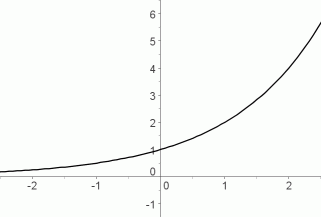increasing functions;
also called growth functions

$\,y=b^x\,$  for  $\,0\lt b\lt 1\,$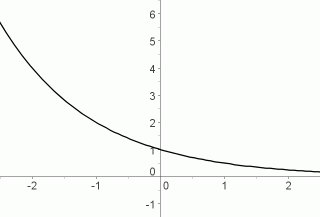decreasing functions;
also called decay functions

## Properties of the Graph for $\,b\gt 1\,$

An increasing function has the following property: as you walk along the graph, going from left to right, you are always going uphill.

The following are equivalent for a function $\,f(x)=b^x\,$:

• $f\,$ is an increasing function
• $b\gt 1\,$

For increasing exponential functions:

• as $\,x\rightarrow \infty \,,$ $\,y\rightarrow \infty \,$
Read this aloud as:  as $\,x\,$ goes to infinity, $\,y\,$ goes to infinity
• as $\,x\rightarrow -\infty \,,$ $\,y\rightarrow 0\,$
Read this aloud as:  as $\,x\,$ goes to negative infinity, $\,y\,$ approaches zero

It is important to note that increasing exponential functions increase VERY quickly.

For example, suppose you are walking along the graph of $\,y={\text{e}}^x\,,$ moving from left to right. By the time $\,x\,$ is $\,7\,,$ the outputs are already greater than $\,1000\,.$

Even more impressive is this fact: as you pass through the point that has $y$-value $\,1000\,,$ the slope of the tangent line is also $\,1000\,,$ so the outputs are changing $\,1000\,$ times faster than the inputs at this point! It would feel like you are climbing a vertical cliff (see below)! (Now, repeat this paragraph with the number $\,1000\,$ replaced by any large number of your choosing!)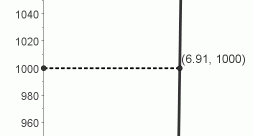$y = b^x\,$   for   $\,b \gt 1$
increasing functions
as $\,x\rightarrow \infty \,,$ $\,y\rightarrow \infty \,$
as $\,x\rightarrow -\infty \,,$ $\,y\rightarrow 0\,$

## Properties of the Graph for $\,0\lt b\lt 1\,$

A decreasing function has the following property: as you walk along the graph, going from left to right, you are always going downhill.

The following are equivalent for a function $\,f(x)=b^x\,$:

• $\,f\,$ is a decreasing function
• $\,b\,$ is between $\,0\,$ and $\,1\,$

For decreasing exponential functions:

• as $\,x\rightarrow \infty \,,$ $\,y\rightarrow 0\,$
Read this aloud as:  as $\,x\,$ goes to infinity, $\,y\,$ approaches zero
• as $\,x\rightarrow -\infty \,,$ $\,y\rightarrow \infty \,$
Read this aloud as:  as $\,x\,$ goes to negative infinity, $\,y\,$ goes to infinity$y = b^x\,$  for  $\,0 \lt b \lt 1$
decreasing functions
as $\,x\rightarrow \infty \,,$ $\,y\rightarrow 0 \,$
as $\,x\rightarrow -\infty \,,$ $\,y\rightarrow \infty\,$

## Properties that All Exponential Functions Share

Let $\,f(x)=b^x\,,$ where $\,b\,$ is a positive number not equal to $\,1\,.$

For all (allowable) bases $\,b\,,$ exponential functions share the following properties:

### The Domain is the Set of All Real Numbers:$\text{dom}(f) = \mathbb{R}$

If the graph of an exponential function is ‘collapsed’ into the $x$-axis, sending each point on the graph to its $x$-value, then the entire $x$-axis will be hit.

Exponential functions know how to act on all real number inputs.

For basic information on the domain and range of a function, you may want to review: Domain and Range of a Function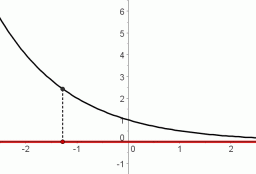### The Range is the Set of All Positive Real Numbers:$\text{ran}(f)=(0,\infty )$

If the graph of an exponential function is ‘collapsed’ into the $y$-axis, sending each point on the graph to its $y$-value, then all positive $y$-values will be hit.

Outputs from exponential functions are always positive.

Having trouble understanding the expression ‘$\,(0,\infty)\,$’ ? Then, you may want to review Interval and List Notation.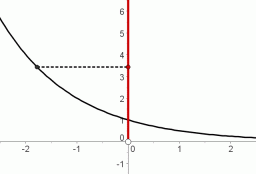### The Graph Crosses the $y$-Axis at $\,y=1\,$

For allowable values of $\,b\,,$   $\,b^0 \ \overset{\text{always}}{\ \ =\ \ }\ 1\,.$  So, when the input is $\,0\,$ to the function $\,y=b^x\,,$ the output is $\,1\,.$ Thus, the point $\,(0,1)\,$ lies on the graph of every exponential function.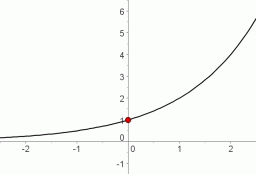### The Graph Passes Both the Vertical and Horizontal Line Tests

Vertical Line Test:  Imagine a vertical line sweeping through a graph, checking each allowable $x$-value: if it never hits the graph at more than one point, then the graph is said to pass the vertical line test.

All functions pass the vertical line test, since the function property is that each input has exactly one output.

passes the vertical line test:
each $x$-value has only one $y$-valueall functions
pass the vertical line test

fails the vertical line test:
there exists an $x$-value
that has more than one $y$-value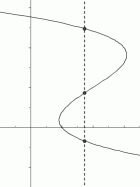Horizontal Line Test:   Imagine a horizontal line sweeping through a graph, checking each allowable $y$-value. If it never hits the graph at more than one point, then the graph is said to pass the horizontal line test. Some functions pass the horizontal line test, and some do not.

passes the horizontal line test:
each $y$-value has only one $x$-value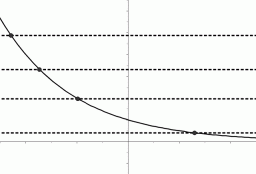all exponential functions
pass the horizontal line test

fails the horizontal line test:
there exists a $y$-value
that has more than one $x$-value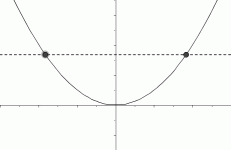some functions
fail the horizontal line test

Thus, exponential functions have a wonderful property: each input has exactly one output (passes the vertical line test), and each output has exactly one input (passes the horizontal line test).

For such functions, you can think of the inputs/outputs as being connected with strings: pick up any input, and follow its ‘string’ to the unique corresponding output; pick up any output, and follow its ‘string’ to the unique corresponding input. That is, there is a one-to-one correspondence between the inputs and outputs. Functions with this property are called one-to-one functions.